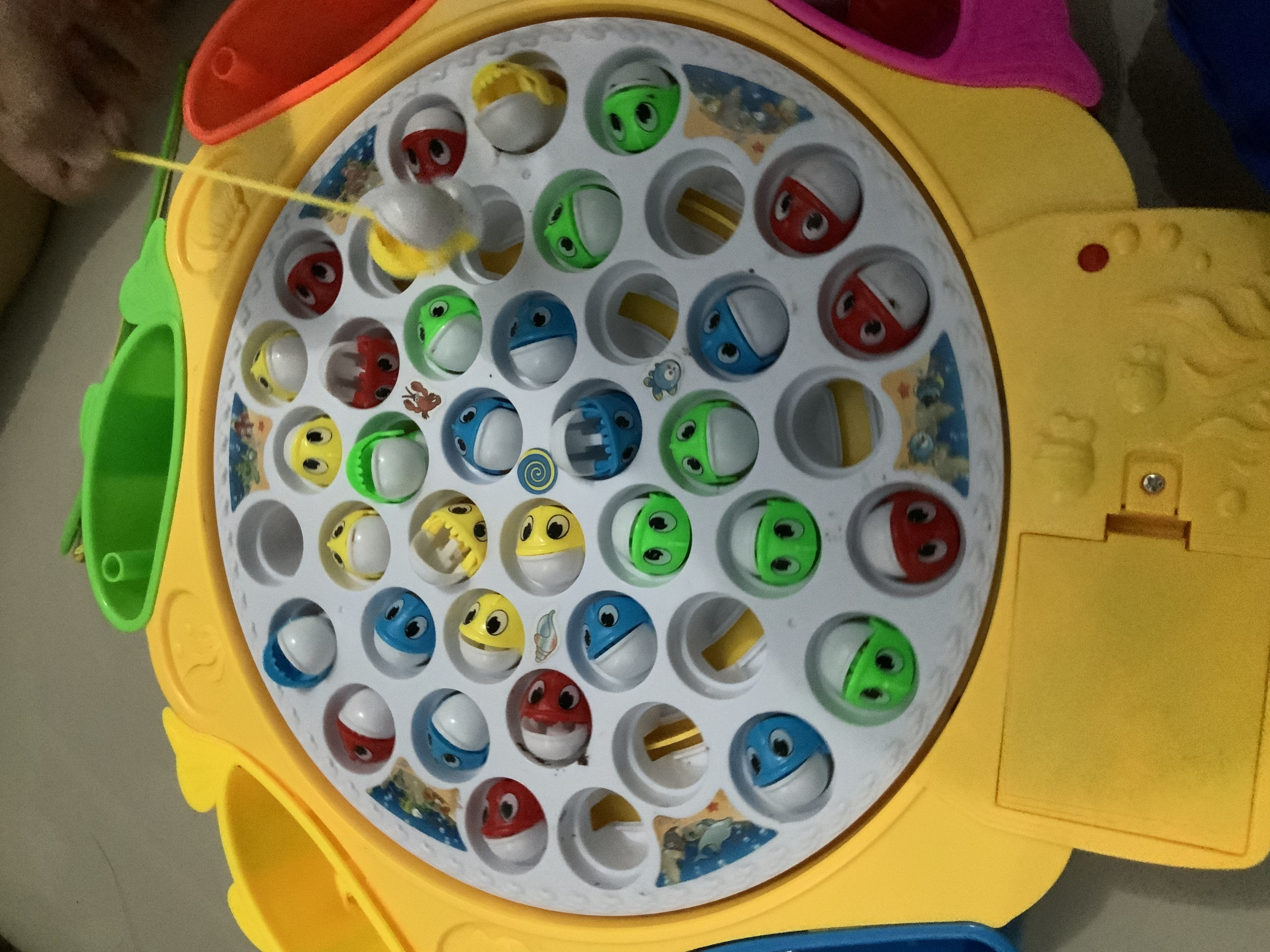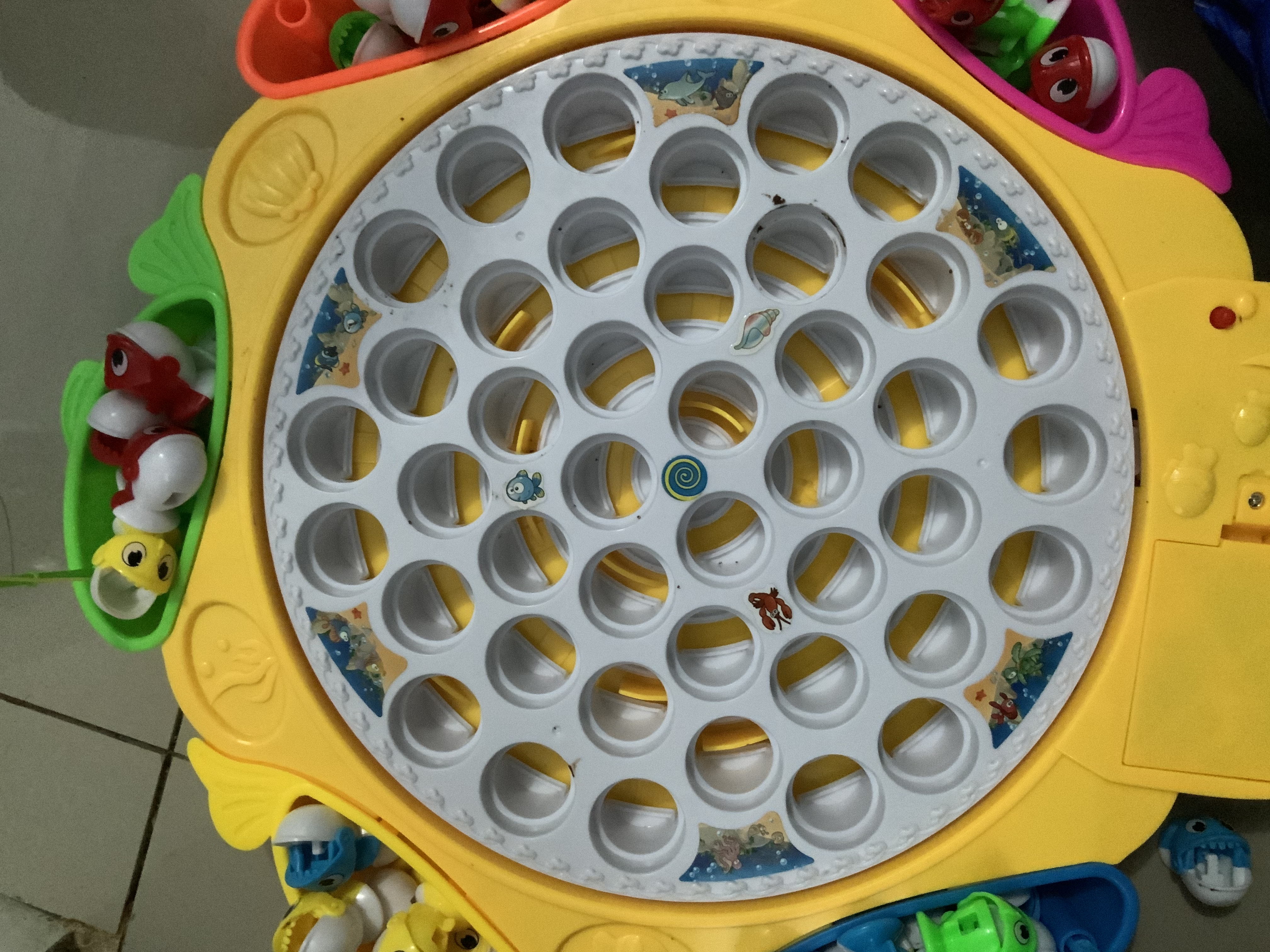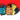Maulana's personal blog
Main feedMath/PhysicsSoftware Development

11-Apr-2021

# A brief look at the toy

I recently bought my daughter a fishing toy.

It looks like this:It’s just a spinning disc with little fishes in it. These fishes sometimes open their mouth, during which you can put hook from the given fishing rod. If the timing is right, you can hook them out of the disc.

The rule is pretty simple, but to actually do it is surprisingly difficult. I began to wonder if the fish location relative to the center of the disc corresponds to the difficulty to hook them out. It took me a couple of attempts to try to hook out the fish at the edges.

Once my daughter and I fished them all out, we reveal the mechanism behind the contraption:If you look closely, there were circular/angular lanes behind the rotating disc. In the lane, there were ramps. These fish will eventually “walk” the ramp, like a train. When they walk the ramp, the fish protrude slightly up, so the mouth opens and you can hook them out. That’s the secret behind this device.

It turns out the ramp have almost similar circular/angular/arc length regardless of the distance from the center. This could be the factor that leads to different difficulties of fishing them out.

# Information we have so far

The disc is spinning with constant angular velocity $\omega$.

There are multiple lanes, characterized by it’s distance from the center. $L(r)$. With $r$ being the distance from the center.

However, in each lane, we assume that the ramp circular/arc distance is all equal to $d$.

We define the difficulty to be $H$. The difficulty possibly relates with how much time the mouth stays open while walking the ramp $t$. So, $H(t)$ is some function of $t$. More difficult to hook out fish with bigger $H(t)$ value.

# Quantifying the difficulties

It’s a simple problem.

For such lane $L(r)$, we have constant angular velocity $\omega$ and ramp length $d$. But the time for the fish in that lane to travel the ramp length is related with the tangential velocity $v$ of the fish. The relationship between angular and tangential velocity:

$v = \omega r$

Tangential velocity is of course the speed at which the fish travel the arc length of the ramp:

$v = \frac{d}{t} = \omega r \\ t = \frac{d}{\omega r}$

By our definition above, the difficulty of hooking out a fish in particular lane $L(r)$ is inversely proportional to $t$. That means:

$H = \frac{\omega r}{d}$

As you can see, our initial hypothesis is correct. The further the fish from the center, the more difficult for us to hook it.

So, fish near the center is easier to hook because it’s mouth open most of the time. :DWritten by Rizky Maulana Nugraha
Software Developer. Currently remotely working from Indonesia.